# AP Physics 1 Multiple-select Practice Test 5

### Test Information9 questions18 minutes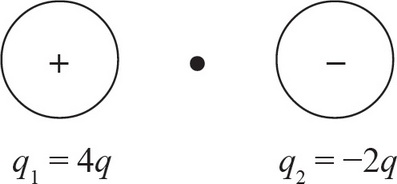1. Consider two small spheres, one carries a charge of q1 = +4 q and the other a charge of q2 = -2 q, separated by a distance of x. Which of the following changes can double the magnitude of the electric field at the midpoint between the two charges? Select two answers.

2. For which combination of springs will the effective spring constant be equal to 2k ? Select two answers.

3. Which of the following must be true for an object at translational equilibrium? Select two answers.

4. Which of the following changes if implemented would increase the frequency of the oscillations of a simple pendulum? Select two answers.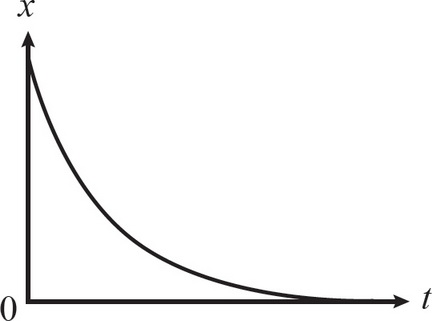5. The graph above is the position-versus-time graph of an object. Which of the following is true regarding the motion of the object? Select two answers.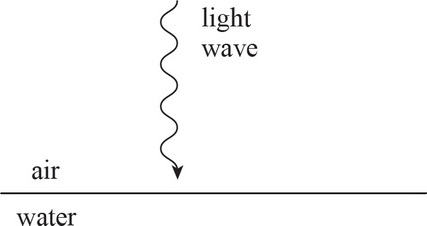6. When a light wave passes from air into water, what properties of the wave change? Select two answers.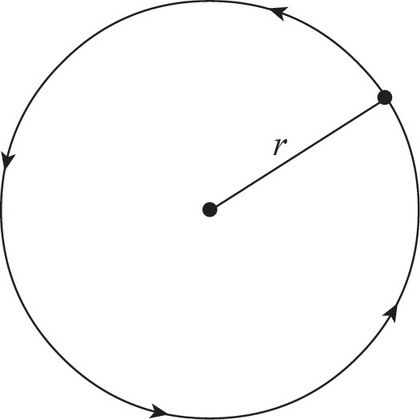7. Which of the following statements is true regarding an object undergoing uniform circular motion? Select two answers.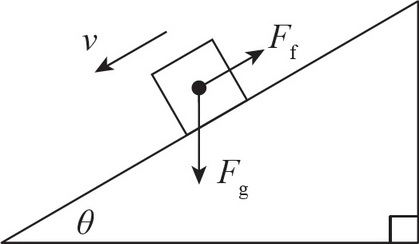8. Which of the following is true regarding conservative forces? Select two answers.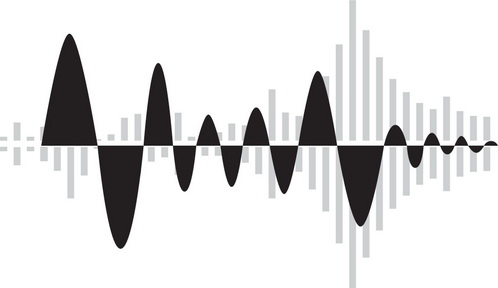9. What does the speed of a wave depend on? Select two answers.